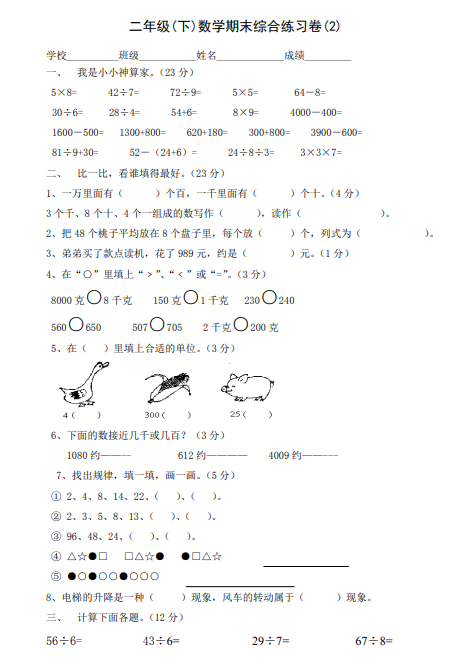ceomax主题已启用，当前站点还没有验证正版主题授权，暂不可使用 前往授权激活 获取正版授权
【PDF文档3页】新人教版小学二年级下册数学期末试卷 (3)A4电子版资料_可直接打印_会员免费下载 - 悟思学_领悟_思考_学习

【PDF文档3页】新人教版小学二年级下册数学期末试卷 (3)A4电子版资料_可直接打印_会员免费下载

761

5×8= 42÷7= 72÷9= 5×5= 64－8=
30÷6= 28÷4= 54+6= 8×9= 4000－400=
1600－500= 1300+800= 620+180= 300+800= 3900－600=
81÷9+30= 52－（24+6）= 24÷8÷3= 3×3×7=

【PDF文档3页】新人教版小学二年级下册数学期末试卷 (3)A4电子版资料_可直接打印_会员免费下载相关资料206 5244 53842

• 0 +

访问总数

• 0 +

会员总数

• 0 +

文档总数

• 0 +

今日发布

• 0 +

本周发布

• 0 +

运行天数